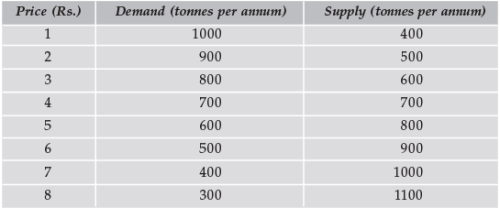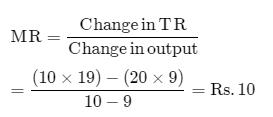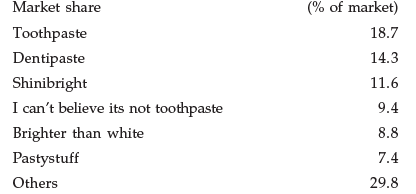Courses

# Test: Price Determination In Different Markets - 1

## 40 Questions MCQ Test Economics for CA CPT | Test: Price Determination In Different Markets - 1

Description
This mock test of Test: Price Determination In Different Markets - 1 for CA Foundation helps you for every CA Foundation entrance exam. This contains 40 Multiple Choice Questions for CA Foundation Test: Price Determination In Different Markets - 1 (mcq) to study with solutions a complete question bank. The solved questions answers in this Test: Price Determination In Different Markets - 1 quiz give you a good mix of easy questions and tough questions. CA Foundation students definitely take this Test: Price Determination In Different Markets - 1 exercise for a better result in the exam. You can find other Test: Price Determination In Different Markets - 1 extra questions, long questions & short questions for CA Foundation on EduRev as well by searching above.
QUESTION: 1

### In the table below what will be equilibrium market price?Solution:

At the equilibrium level, market demand is equal to market supply. In the above schedule at the price level of Rs. 4, market demand = market supply = 700

QUESTION: 2

### Assume that when price is Rs. 20, quantity demanded is 9 units, and when price is Rs. 19, quantity demanded is 10 units. Based on this information, what is the marginal revenue resulting from an increase in output from 9 units to 10 units.

Solution:QUESTION: 3

### Assume that when price is Rs.20, quantity demanded is 15 units, and when price is Rs.18, quantity demanded is 16 units. Based on this information, what is the marginal revenue resulting from an increase in output from 15 units to 16 units?

Solution:
QUESTION: 4

Suppose a firm is producing a level of output such that MR > MC. What should be firm do to maximize its profits?

Solution:
QUESTION: 5

Marginal Revenue is equal to :

Solution:
QUESTION: 6

Suppose that a sole proprietorship is earning total revenues of Rs. 1,00,000 and is incurring explicit costs of Rs. 75,000. If the owner could work for another company for Rs. 30,000 a year, we would conclude that :

Solution:
QUESTION: 7

Which of the following is not an essential condition of pure competition?

Solution:
QUESTION: 8

What is the shape of the demand curve faced by a firm under perfect competition?

Solution:
QUESTION: 9

Which is the first order condition for the profit of a firm to be maximum?

Solution:
QUESTION: 10

Which of the following is not a characteristic of a “price taker”?

Solution:
QUESTION: 11

Which of the following statements is false?

Solution:
QUESTION: 12

With a given supply curve, a decrease in demand causes

Solution:
QUESTION: 13

It is assumed in economic theory that

Solution:
QUESTION: 14

Assume that consumers’ incomes and the number of sellers in the market for good A both decrease. Based upon this information we can conclude, with certainty, that equilibrium :

Solution:
QUESTION: 15

Suppose that the supply of cameras increases due to an increase in foreign imports. Which of the following will most likely occur?

Solution:
QUESTION: 16

Assume that in the market for good Z there is a simultaneous increase in demand and the quantity supplied. The result will be :

Solution:
QUESTION: 17

Suppose the technology for producing personal computers improves and, at the same time, individuals discover new uses for personal computers so that there is greater utilisation of personal computers. Which of the following will happen to equilibrium price and equilibrium quantity?

Solution:
QUESTION: 18

Which of the following is not a condition of perfect competition?

Solution:
QUESTION: 19

Which of the following is not a characteristic of a perfectly competitive market?

Solution:
QUESTION: 20

Which of the following is not a characteristic of monopolistic competition?

Solution:
QUESTION: 21

All of the following are characteristics of a monopoly except :

Solution:
QUESTION: 22

Oligopolistic industries are characterized by :

Solution:
QUESTION: 23

Price-taking firms, i.e., firms that operate in a perfectly competitive market, are said to be “small” relative to the market. Which of the following best describes this smallness?

Solution:
QUESTION: 24

For the price-taking firm :

Solution:
QUESTION: 25

Monopolistic competition differs from perfect competition primarily because

Solution:
QUESTION: 26

The long-run equilibrium outcomes in monopolistic competition and perfect competition are similar, because in both market structures

Solution:
QUESTION: 27

A monopolist is able to maximise his profits when :

Solution:
QUESTION: 28

In which form of the market structure is the degree of control over the price of its product by a firm very large?

Solution:
QUESTION: 29

​Which is the other name that is given to the average revenue curve?

Solution:
QUESTION: 30

Under which of the following forms of market structure does a firm have no control over the price of its product?

Solution:
QUESTION: 31

Discriminating monopoly implies that the monopolist charges different prices for his commodity :

Solution:
QUESTION: 32

Price discrimination will be profitable only if the elasticity of demand in different market in which the total market has been divided is :

Solution:
QUESTION: 33

The Kinked demand hypothesis is designed to explain in the context of oligopoly

Solution:
QUESTION: 34

The firm in a perfectly competitive market is a price taker. This designation as a price taker is based on the assumption that

Solution:
QUESTION: 35

Suppose that the demand curve for the XYZ Co. slopes downward and to the right. We can conclude that

Solution:
QUESTION: 36

If firms in the toothpaste industry have the following market shares, which market structure would best describe the industry?Solution:
QUESTION: 37

The kinked demand curve model of oligopoly assumes that

Solution:

The kinked demand curve model of oligopoly assumes that response to a price increase is less than the response to a price decrease. In an oligopolistic market, the kinked demand curve hypothesis states that the firm faces a demand curve with a kink at the prevailing price level. The curve is more elastic above the kink and less elastic below it. This means that the response to a price increase is less than the response to a price decrease.

QUESTION: 38

A firm encounters its “shutdown point” when :

Solution:
QUESTION: 39

Suppose that, at the profit-maximizing level of output, a firm finds that market price is less than average total cost, but greater than average variable cost. Which of the following statements is correct?

Solution:
QUESTION: 40

When price is less than average variable cost at the profit-maximising level of output, a firm should :

Solution: